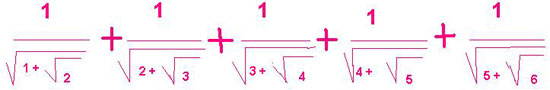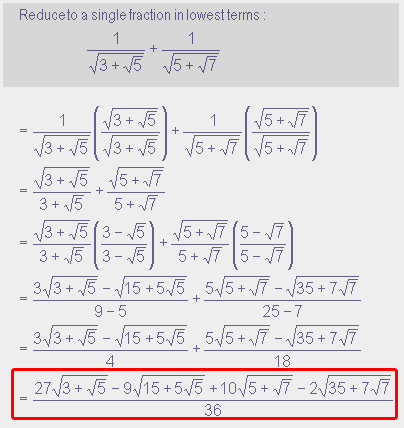SEARCH HOMEMath Central Quandaries & QueriesHow do I simplify this expression with surds in the denominator? INDRAJIT INDIAHello Indrajit.

Here is the problem you sentYou will need to find a common denominator: one that has no radicals in it.

So for each fraction:
1. multiply the numerator and the denominator by the denominator. That will remove the first radical in the inner one and leave the second.
2. multiply the numerator and the denominator by the conjugate of the denominator. Because the conjugate gives you the difference of squares, this removes the other radical in the denominator (but yes, your numerator will look terrible now).

Finally, find a common denominator as you do for all fraction additions and simplify the numerator as much as you can (if you can at all).

Here is an example, simpler than the one you need to do, but you can see that the numerator gets pretty complex:Cheers,
Stephen La Rocque.Math Central is supported by the University of Regina and The Pacific Institute for the Mathematical Sciences.